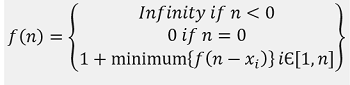Q:

# Get Minimum Squares

Given a number N, write a program to print the minimum number of squares that sums to N.

```    Input:
N = 41

Output:
Minimum number of squares needed: 2
```

Explanation with example

We can think similar way as the coin change problem (refer to my article on coin change). We can start with the greedy approach and can see whether it works or not.

So, a greedy approach can be

First thing is to find whether we will be able to find answers for any number N. Is it possible to find squares that will sum up to N. Yes, we will be able to find for any number N because 1 is itself a square and we can get any number by summing 1?

Since we are to find the minimum number of squares it's apparent that we should pick the square with the maximum value closest to N and keep trying with that until the remaining sum is less the square. Then on course, we keep choosing the next best one. So, the algorithm would be like.

```    1) Let, count=0 to count minimum number of squares to sum
2) Pick up square x closest to N
3)  While N≥x
N=N-x
count=count+1

4)  if N=0
Go to Step 7
5) Pick up the next best square value <x & closest to N .assign it to x
6) Go to Step 2
7) End. count is the minimum number of squares needed to sum up.
```

Let's see whether the above greedy algorithm leads to the desired result or not.

```    N = 41
The square value closest to 41 is 36
count = 1, N = 5
Next best square value is 4
count = 2, N = 1
Next best square value is 1
count = 3, N = 0
So, minimum number of squares needed is 3
But is it really minimum?
If we choose 25 and 16, that sums up to 41 and we need only two squares.
That's the minimum, not 3.
So, greedy does not work as the local optimum choices doesn't make
global optimum choice. Hence, we need dynamic programing.```

In the coin change problem (refer to my article of coin change) we had an amount M and n number of coins, { C1, C2, ..., Cn} to make change. We can think this minimum square problem as we did in coin change problem. We can frame this problem lie the coin change problem, where, Amount M = Number N

Coin denominations { C1, C2, ..., Cn} = {x1, x2, ..., xn } where xi is square and xi<N

Now,

We have two choice for any xi,

1. Use xi and recur for N-xi
2. Don't use xi and recur for N with other squares

f(n)= minimum number of squares needed to sum nWe can formulate the above recursion using DP.

```    1) Initialize DP[N+1] with index value
[for any number 0 to N, minimum number of squares needed
is initially same as index no, i.e., minimum number squares
needed for number i=i( i times 1) ]
2) DP=0 //base case of above recursion
3) Create X[n]with squares<N
4)  for  i=1 to N //iterate numbers
for squares xi = X  to X[n]
If i>=xi && DP[i-xi]+1<DP[i])
DP[i]=DP[i-xi]+1; //update value
End for
End for

The result is DP[N]
```

C++ implementation:

``````#include <bits/stdc++.h>
using namespace std;

int min_square(int m)
{

int DP[m + 1];
//base value
for (int i = 1; i <= m; i++)
DP[i] = i;
DP = 0;

//create the array of squares to be used to sum
vector<int> a;
for (int i = 1;; i++) {
if (i * i <= m)
a.push_back(i * i);
else
break;
}
int n = a.size();
for (int i = 1; i <= m; i++) { //iterate for the numbers
for (int j = 0; j < n; j++) { //iterate on the squares
if (i >= a[j] && DP[i - a[j]] + 1 < DP[i])
DP[i] = DP[i - a[j]] + 1;
}
}

//result
return DP[m];
}

int main()
{
int n, item, m;

cout << "Enter the number\n";
cin >> n;

int ans = min_square(n);
cout << "Minimum number of squares needed: " << ans << endl;

return 0;
}
``````

Output

```Enter the number
41
Minimum number of squares needed: 2```